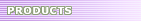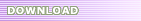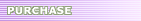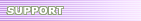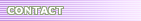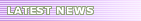# Ellemtel C++ Style and Rules - Type Conversions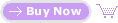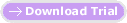13. Type Conversions Rule 43Never use explicit type conversions (casts). Rule 44Do not write code which depends on functions that use implicit type conversions. Rule 45Never convert pointers to objects of a derived class to pointers to objects of a virtual base class. Rule 46Never convert a `const` to a non-`const`. A type conversion may either be explicit or implicit, depending on whether it is ordered by the programmer or by the compiler. Explicit type conversions (casts) are used when a programmer want to get around the compiler's typing system; for success in this endeavour, the programmer must use them correctly. Problems which the compiler avoids may arise, such as if the processor demands that data of a given type be located at certain addresses or if data is truncated because a data type does not have the same size as the original type on a given platform. Explicit type conversions between objects of different types lead, at best, to code that is difficult to read. Explicit type conversions (casts) can be motivated if a base class pointer to a derived class pointer is needed. This happens when, for example, a heterogeneous container class is used to implement a container class to store pointers to derived class objects. This new class can be made "type-safe" if the programmer excludes other objects than derived class pointers from being stored. In order for this implementation to work, it is necessary that the base class pointers are converted to derived class pointers when they are removed from the heterogeneous container class. The above reason for using explicit casts will hopefully disappear when templates are introduced into C++. It is sometimes said that explicit casts are to object-oriented programming, what the `goto` statement was to structured programming. There are two kinds of implicit type conversions: either there is a conversion function from one type to another, written by the programmer, or the compiler does it according to the language standard. Both cases can lead to problems. C++ is lenient concerning the variables that may be used as arguments to functions. If there is no function which exactly matches the types of the arguments, the compiler attempts to convert types to find a match. The disadvantage in this is that if more than one matching function is found, a compilation error will be the result. Even worse is that existing code which the compiler has allowed in other contexts, may contain errors when a new implicit type conversion is added to the code. Suddenly, there may be more than one matching function. [See Example 53!] Another unpredictable effect of implicit type conversions is that temporary objects are created during the conversion. [See Example 51!] This object is then the argument to the function; not the original object. The language definition forbids the assignment of temporary objects to non-constant references, but most compilers still permit this. In most cases, this can mean that the program does not work properly. Be careful with constructors that use only one argument, since this introduces a new type conversion which the compiler can unexpectedly use when it seems reasonable in a given situation. Virtual base classes give rise to other type conversion problems. It is possible to convert a pointer, to an instance of a class which has a virtual base class, to a pointer to an object of that virtual base class. The opposite conversion is not allowed, i.e. the type conversion is not reversible. For this reason, we do not recommend the conversion of a derived class pointer to a virtual base class pointer. In order to return a non-const temporary object, it sometimes happens that an explicit type conversion is used to convert const member data to non-const. This is bad practice that should be avoided, primarily because it should be possible for a compiler to allocate constants in ROM (Read Only Memory). [See Example 54 and Example 55.] Exception to Rule 43An explicit type conversion (cast) is preferable to a doubtful implicit type conversion. Explicit type conversions may be used to convert a pointer to a base class to a pointer of a derived class within a type-safe container class that is implemented using a heterogeneous container class. Explicit type conversion must be used to convert an anonymous bit-stream to an object. This situation occurs when unpacking a message in a message buffer. Generally, explicit type conversions are needed for reading an external representation of an object. Exception to Rule 44At times it is desirable to have constructors that use only one argument. By performing an explicit type conversion, the correctness of the code does not depend on the addition. See the Exception to Rule 22! Exception to Rule 45If a virtual base class is to contain a pure virtual function which converts a virtual base class pointer to a derived class pointer, this can be made to work by defining the function in the derived class. Note that this implies that all derived classes must be known in the virtual base class. See Example 52! Exception to Rule 46No exceptions. Use pointers to data allocated outside the class, when necessary. See Example 54 and Example 55. Example 49: Constructors with a single argument that may imply dangerous type conversions ``` class String { public: String( int length ); // Allocation constructor // ... }; // Function that receives an object of type String as an argument void foo( const String& aString ); // Here we call this function with an int as argument int x = 100; foo( x ); // Implicit conversion: foo( String( x ) ); ``` Example 50: A use of implicit type conversion ``` // String.hh class String { public: String( char* cp ); // Constructor operator const char* () const; // Conversion operator to const char* // ... }; void foo( const String& aString ); void bar( const char* someChars ); // main.cc main() { foo( "hello" ); // Implicit type conversion char* -> String String peter = "pan"; bar( peter ); // Implicit type conversion String -> const char* } ``` Example 51: When implicit type conversion gives unpleasant results ``` // This function looks bulletproof, but it isn't. // Newer versions of compilers should flag this as an error. void mySwap( int& x, int& y ) { int temp = x; x = y; y = temp; } int i = 10; unsigned int ui = 20; mySwap( i, ui ); // What really happens here is: // int T = int( ui ); // Implicit conversion // mySwap( i, T ); // ui is of course not changed! // Fortunately, the compiler warns for this ! ``` Example 52: Conversion of derived class pointer to a virtual base class pointer is irreversible ``` class VirtualBase { public: virtual class Derived* asDerived() = 0; }; class Derived : virtual public VirtualBase { public: virtual Derived* asDerived(); }; Derived* Derived::asDerived() { return this; } void main() { Derived d; Derived* dp = 0; VirtualBase* vp = (VirtualBase*)&d; dp = (Derived*)vp; // ERROR! Cast from virtual base class pointer dp = vp->asDerived(); // OK! Cast in function asDerived } ``` Example 53: Addition which leads to a compile-time error ``` // String.hh class String { public: String( char* cp ); // Constructor operator const char* () const; // Conversion operator to const char* // ... }; void foo( const String& aString ); void bar( const char* someChars ); // Word.hh class Word { public: Word( char* cp ); // Constructor // ... }; // Function foo overloaded void foo( const Word& aWord ); // ERROR: foo( "hello" ) MATCHES BOTH: // void foo( const String& ); // AND void foo( const Word& ); //main.cc main() { foo( "hello" ); // Error ambiguous type conversion ! String peter = "pan"; bar( peter ); // Implicit type conversion String -> const char* } ``` Example 54: For more efficient execution, remove const-ness when storing intermediate results ``` // This is code is NOT recommended #include class Vector { public: Vector(int, const int []); // Constructor double length() const; // length = sqrt(array*array + ... ) void set(int x, int value); // ... private: int size; int* array; double lengthCache; // to cache calculated length int hasChanged; // is it necessary to re-calculate length ? }; double Vector::length() const { if (hasChanged) // Do we need to re-calculate length { ((Vector*)this)->hasChanged=0; // No! Cast away const double quadLength = 0; for ( int i = 0; i < size; i++ ) { quadLength += pow(array[i],2); } ((Vector*)this)->lengthCache = sqrt(quadLength); // No! Cast away const } return lengthCache; } void Vector::set( int nr, int value ) { if ( nr >= size ) error( "Out Of Bounds"); array[nr]=value; hasChanged = 1; } ``` Example 55: Alternative to removing const-ness for more efficient execution ``` // This is code is safer than Example 54 but could be inefficient #include class Vector { public: Vector(int, const int []); // Constructor double length() const; // length = sqrt(array*array + ... ) void set(int x, int value); // ... private: int size; int* array; double* lengthCache; // to cache length in int* hasChanged; // is it necessary to re-calculate length ? }; Vector::Vector(int sizeA, const int arrayA[]) : size(sizeA), array( new int[sizeA] ), hasChanged(new int(1)), lengthCache(new double) { for ( int i = 0; i < size; i++ ) { array[i] = arrayA[i]; } } Vector::~Vector() // Destructor { delete array; delete hasChanged; delete lengthCache; } // Continue on next page ! double Vector::length() const { if (hasChanged) // Do we need to re-calculate length ? { *hasChanged=0; double quadLength = 0; for ( int i = 0; i < size; i++ ) { quadLength += pow(array[i],2); } *lengthCache = sqrt(quadLength); } return lengthCache; } void Vector::set( int nr, int value ) { if ( nr >= size ) error( "Out Of Bounds"); array[nr]=value; *hasChanged = 1; } ```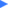Want to align the types of C++ code rapidly?  Use SourceFormatX C++ Code Beautifier to beautify your C++ source code files.